Symmetry Definition

Symmetry
Serial order wise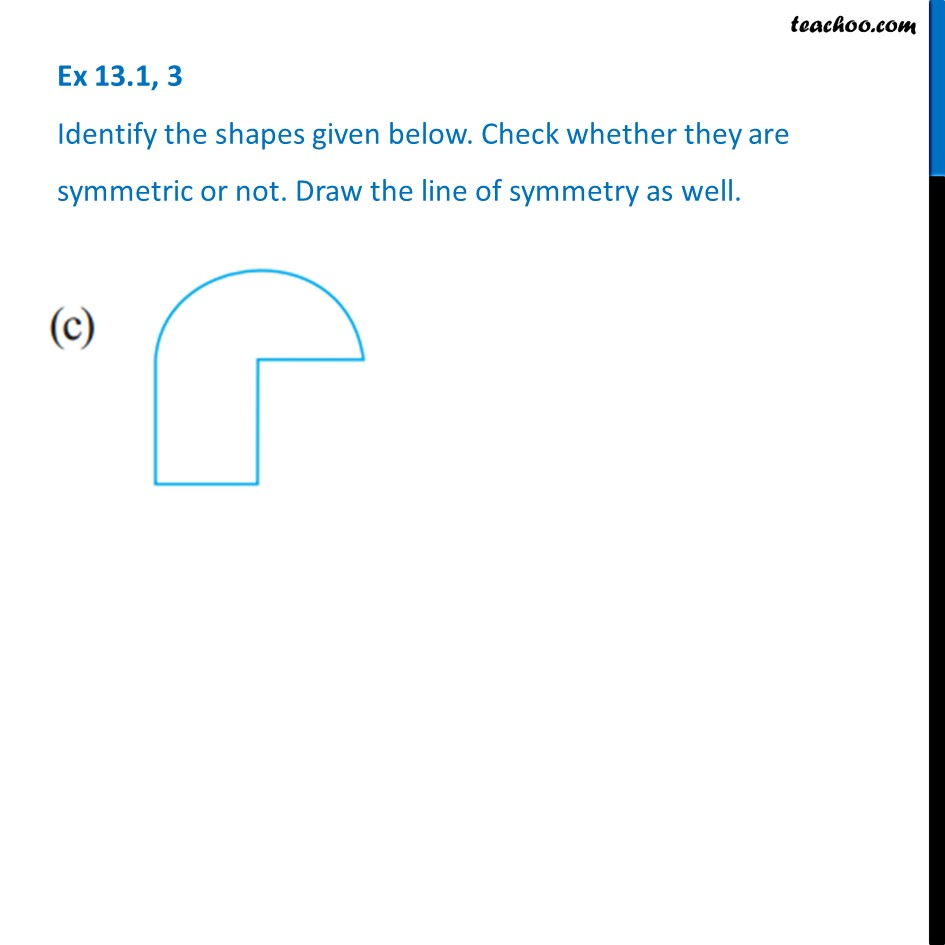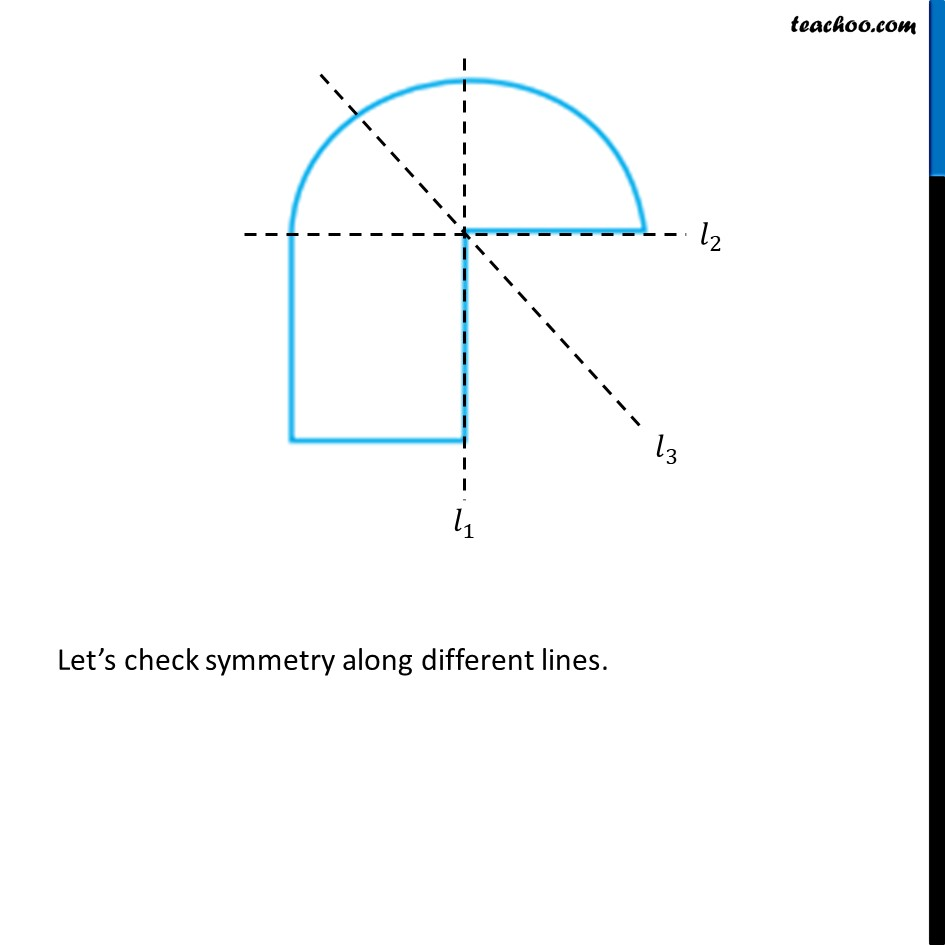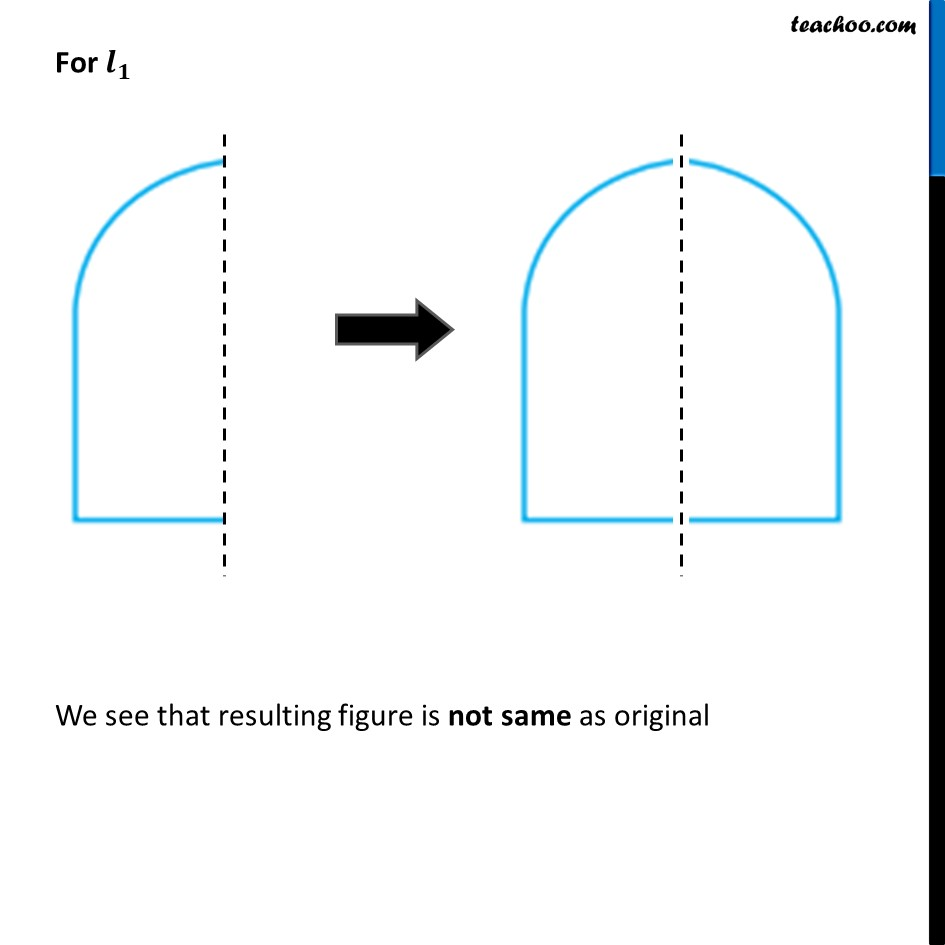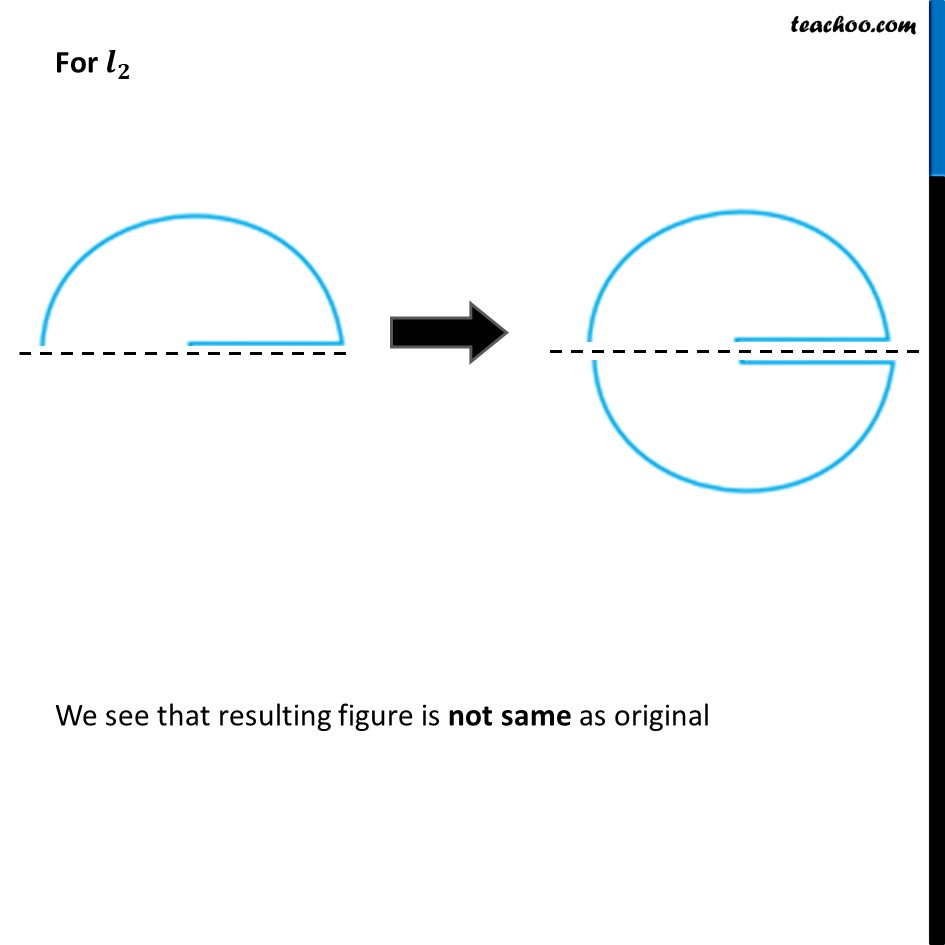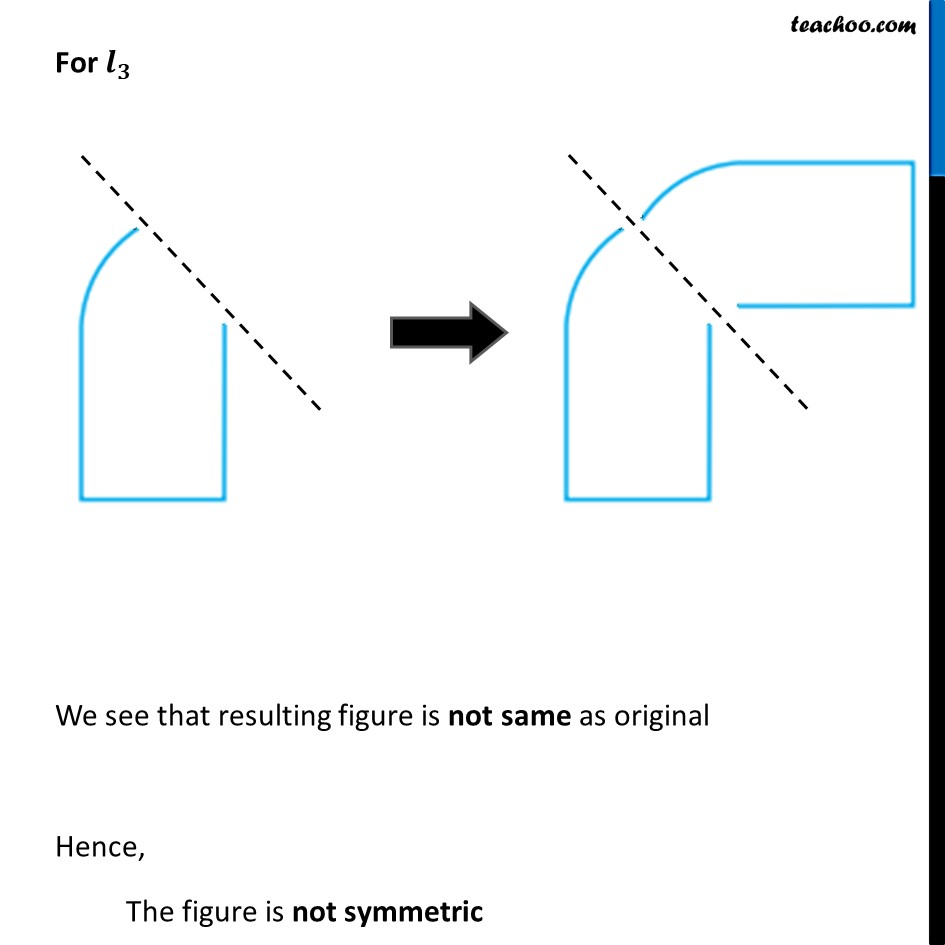Learn in your speed, with individual attention - Teachoo Maths 1-on-1 Class

### Transcript

Question 3 Identify the shapes given below. Check whether they are symmetric or not. Draw the line of symmetry as well. In this figure, If we draw a straight line through the center We can see that the figure is symmetric Let’s check So, the figure is symmetric Also, The figure is not symmetric along any other line Then the figure would be